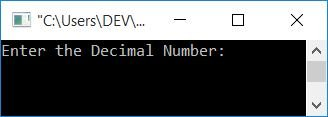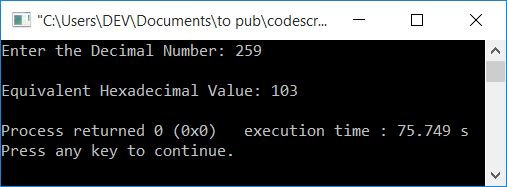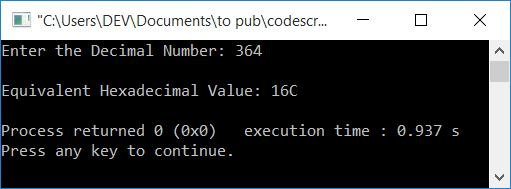# C++ Program to Convert Decimal to Hexadecimal

In this article, you will learn and get code on decimal to hexadecimal conversion in C++. The decimal to hexadecimal conversion program in C++ is created here using the following approaches:

But before going through these programs, if you are not aware of some simple steps used for the conversion, then you can refer to Decimal to Hexadecimal to get all the things you need.

## Decimal to Hexadecimal in C++

To convert a number from decimal to hexadecimal in C++ programming, you have to ask the user to enter the desired decimal number first. and then convert it into its equivalent hexadecimal value. Finally, print its equivalent hexadecimal value on the output as shown in the program given below.

The question is: write a program in C++ to receive a decimal number and print its equivalent value in hexadecimal.  The answer to this question is given below:

```#include<iostream>
using namespace std;
int main()
{
int decimalNum, rem, i=0;
cout<<"Enter the Decimal Number: ";
cin>>decimalNum;
while(decimalNum!=0)
{
rem = decimalNum%16;
if(rem<10)
rem = rem+48;
else
rem = rem+55;
i++;
decimalNum = decimalNum/16;
}
for(i=i-1; i>=0; i--)
cout<<endl;
return 0;
}```

This program was built and runs under the Code::Blocks IDE. Here is its sample run:Now enter the input, say 259 (a decimal number), and press the ENTER key to convert and print its equivalent value in hexadecimal as shown in the snapshot given below:The following is the output of a dry run of the above program with user input of 259 (a decimal number):

• Initial value, i=0
• When the user enters the decimal number input, say 259, then it gets stored in the decimalNum variable. So decimalNum=259
• Now the condition of the while loop gets evaluated.
• That is, the condition decimalNum!=0 or 259!=0 evaluates to be true, therefore program flow goes inside the loop.
• decimalNum%16 or 259%16 or 3 is set to rem. So rem=3
• Now the condition rem<10 or 3<10 evaluates to be true, therefore program flow goes inside the if's body and the statement
`rem = rem+48;`
gets evaluated
• That is, rem+48 or 3+48 or 51 gets initialized to rem. So rem=51
• else's body is skipped because the condition of if evaluates to true.
• Now the value of rem (51) gets initialized to hexaDecimalNum[i] or hexaDecimalNum.
• The value of i gets incremented. Now i=1
• And then decimalNum/16 or 259/16 or 16 gets initialized to decimalNum. So decimalNum=16
• Now program flow goes back and evaluates the condition of the while loop again with a new value of decimalNum (16).
• Again, the condition evaluates to be true, so the program flow again goes inside the loop.
• decimalNum%16 or 16%16 or 0 is assigned to rem.
• Again the if's condition rem<10 or 0<10 evaluates to be true, therefore rem+48 or 0+48 or 48 gets initialized to rem. Now rem=0
• Again, the value of i gets incremented. So i=2
• decimalNum/16 or 1 is set to decimalNum.
• Continue the process until the condition of the while loop evaluates to be false.
• If we continue the process, the value of rem will be 51, 48, and 49 which will be initialized to hexaDecimalNum, hexaDecimalNum, and hexaDecimalNum.
• Print the hexaDecimalNum[] value from the last to the 0th index.
• Because 51, 48, and 49 are ASCII values of 3, 0, and 1, respectively. Therefore, in reverse order, 103 gets printed using the for loop. That is the equivalent hexadecimal value of the given decimal number (that was 259).

Note: If the value of rem (the remainder) is greater than or equal to 10, then the equivalent hexadecimal alphabet's ASCII value (A-F) gets assigned. That is, A for 10, B for 11, C for 12, and so on.

### From 1 to 20, convert decimal to hexadecimal

This program converts all the decimal numbers from 1 to 20 into their equivalent hexadecimal values without asking for user input.

```#include<iostream>
using namespace std;
int main()
{
int decimalNum, rem, i, k;
for(k=1; k<=20; k++)
{
decimalNum=k;
i=0;
while(decimalNum!=0)
{
rem = decimalNum%16;
if(rem<10)
rem = rem+48;
else
rem = rem+55;
i++;
decimalNum = decimalNum/16;
}
cout<<k<<"\t\t";
for(i=i-1; i>=0; i--)
cout<<endl;
}
return 0;
}```

Here is its sample output:### Decimal to hexadecimal conversion in C++, using function

Let's create the same-purpose program using the user-defined function DecToHexDec(). This function converts a decimal number to hexadecimal.

```#include<iostream>
using namespace std;
int DecToHexDec(int dec, int);
int main()
{
int decimalNum, i;
cout<<"Enter the Decimal Number: ";
cin>>decimalNum;
i = DecToHexDec(decimalNum, 0);
for(i=i-1; i>=0; i--)
cout<<endl;
return 0;
}
int DecToHexDec(int dec, int i)
{
int rem;
while(dec!=0)
{
rem = dec%16;
if(rem<10)
rem = rem+48;
else
rem = rem+55;
i++;
dec = dec/16;
}
return i;
}```

Here is a sample run with 364 as a decimal number as user input:#### The same program in different languages

C++ Quiz

« Previous Program Next Program »